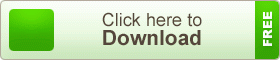# SSC Mathematics Note 7th Chapter Practical Geometry

SSC Mathematics Note 7th Chapter Practical Geometry. In the previous classes, geometrical figures were drawn in proving different propositions and in the exercises. There was no need for precision in drawing these figures.  But sometimes precision is necessary for geometrical constructions.  for example, when an architect makes a design of a house or an engineer draws different parts of a machine, high precision of drawing is required.  In such geometrical constructions, one makes use of ruler and compasses only. We have already learned how to construct triangles and quadrilaterals with the help of ruler and compasses. In this chapter, we will discuss the construction of some special triangles and quadrilaterals.

## SSC Mathematics Note 7th Chapter Practical GeometryEvery triangle has three sides and three angles. But, to specify the shape and size of a triangle, all sides and angles need not be specified. for example, as some of the three angles of a triangle are two right angles, one can easily find the measurement of the third angle when the measurement of the two angles of the triangle given. Again, from the theorems on the congruence of triangles, it is found that the following combination of three sides and angles are enough to be congruent. That is, a combination of these three parts of a triangle is enough to construct a unique triangle.

teachingbd24.com is such a website where you will get all kinds of necessary information regarding educational notes, suggestions and question patterns of schools, colleges, and madrasas. Particularly, you will get here special notes of physics that will be immensely useful to both students and teachers. The builder of the website is Mr. Md. Shah Jamal who has been serving for 32 years as an Assistant Professor of Physics at BAF Shaheen College Dhaka. He expects that this website will meet up all the needs of Bengali version learners /students. He has requested concerned students and teachers to spread this website home and abroad.